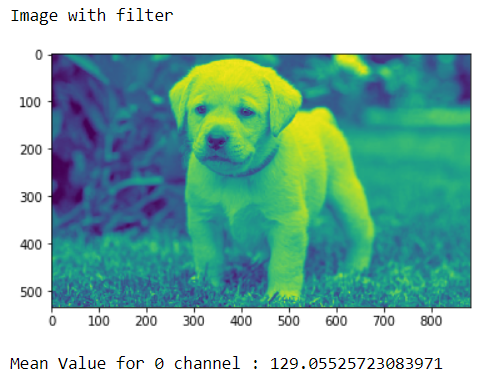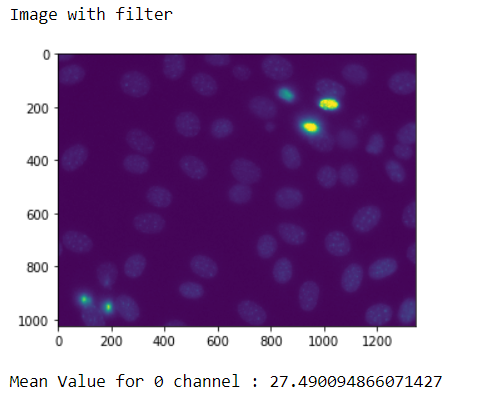Related Articles

# Mahotas – Getting Mean Value of Image

• Last Updated : 16 Apr, 2021

In this article we will see how we can get the mean value of image in mahotas. Mean value is the sum of pixel values divided by the total number of pixel values.
Pixel Values Each of the pixels that represents an image stored inside a computer has a pixel value which describes how bright that pixel is, and/or what color it should be. In the simplest case of binary images, the pixel value is a 1-bit number indicating either foreground or background.
Mean is most basic of all statistical measure. Means are often used in geometry and analysis; a wide range of means have been developed for these purposes. In contest of image processing filtering using mean is classified as spatial filtering and used for noise reduction.
In order to do this we will use mean method

Syntax : img.mean()
Argument : It takes no argument
Return : It returns float32

Here img is the image loaded using mahotas, which can be done with the help of mahotas.imread(image_name) method.
Note : The image should be filtered before getting mean because it can calculate for one channel at one time
Example 1 :

## Python3

 `# importing required libraries``import` `numpy as np``import` `mahotas``from` `pylab ``import` `imshow, show`` ` `# loading image``img ``=` `mahotas.imread(``'dog_image.png'``)`` ` `# filtering the image``img ``=` `img[:, :, ``0``]``   ` `print``(``"Image with filter"``)``# showing the image``imshow(img)``show()`` ` `# getting mean value``mean ``=` `img.mean()`` ` `# printing mean value``print``(``"Mean Value for 0 channel : "` `+` `str``(mean))`

Output :`Mean Value for 0 channel : 129.05525723083971`

Example 2 :

## Python3

 `# importing required libraries``import` `mahotas as mh``import` `mahotas.demos``import` `numpy as np``from` `pylab ``import` `imshow, show` `# getting nuclear image``nuclear ``=` `mh.demos.nuclear_image()`  `# filtering the image``nuclear ``=` `nuclear[:, :, ``0``]` `print``(``"Image with filter"``)``# showing the image``imshow(nuclear)``show()` `# getting mean value``mean ``=` `nuclear.mean()` `# printing mean value``print``(``"Mean Value for 0 channel : "` `+` `str``(mean))`

Output :`Mean Value for 0 channel : 27.490094866071427`

Note : For each channel there are different mean value and mean can be a good option to set the threshold value for an image.

Attention geek! Strengthen your foundations with the Python Programming Foundation Course and learn the basics.

To begin with, your interview preparations Enhance your Data Structures concepts with the Python DS Course. And to begin with your Machine Learning Journey, join the Machine Learning – Basic Level Course

My Personal Notes arrow_drop_up